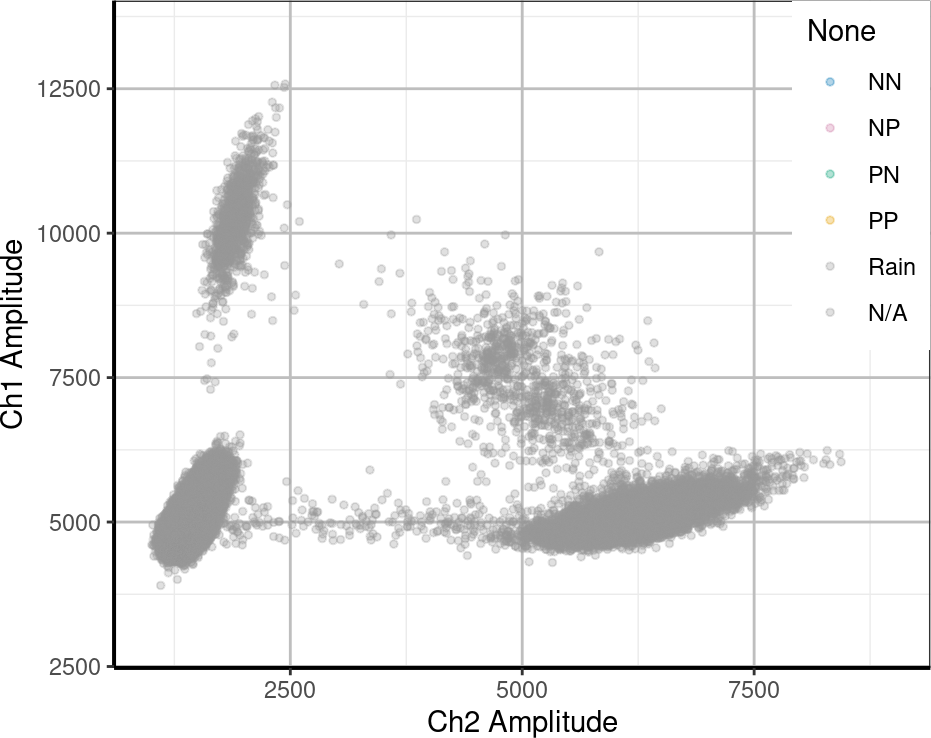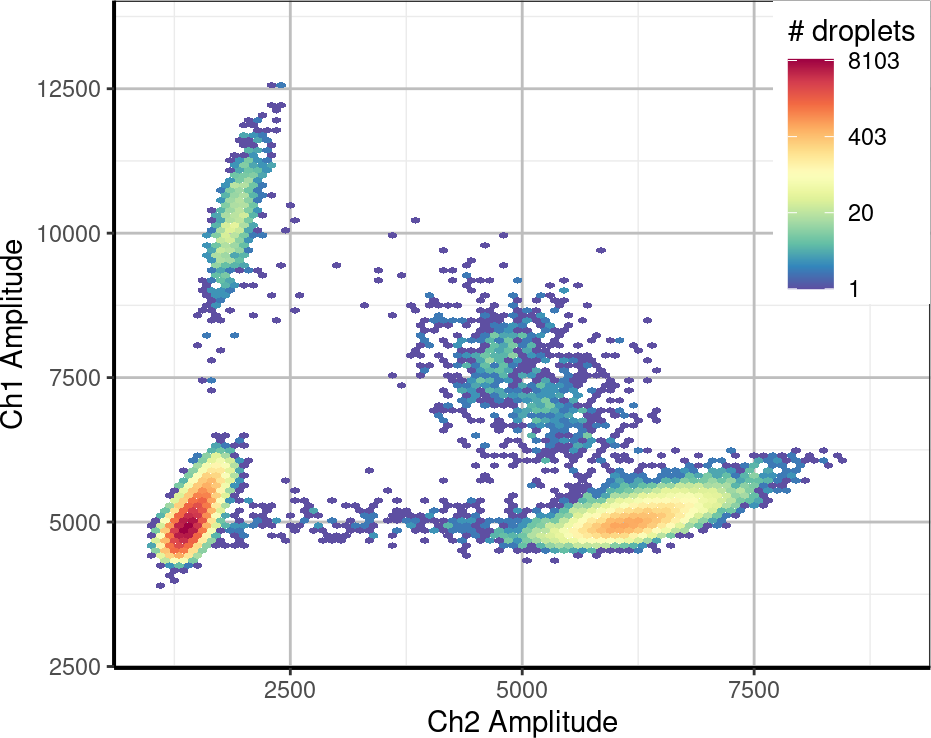# 1 Introduction

Droplet Digital PCR (ddPCR) is a system from Bio-Rad for estimating the number of genomic fragments in samples. ddPCR attaches fluorochromes to targets of interest, for example, mutant and wild type KRAS. Each sample is then divided into 20,000 droplets and qPCR is run on each droplet. The brightness of these droplets is measured in two channels, each corresponding to our targets. The amplitudes of the droplets can be plotted and the results analysed to see whether droplets can be called as:

• Positive in both channels (PP),
• Positive in Channel 1 only (PN),
• Positive in Channel 2 only (NP), or
• Negative in both channels (NN).

There are variations in the brightnesses of the droplets; this can be particularly evident in and around the PP cluster, where there may be some crosstalk due the presence of both fluorochromes. The classification of droplets is therefore not necessarily as simple as deciding on brightness thresholds for each channel, above which a positive reading is called in that channel.

This vignette demonstrates how the `twoddpcr` package may be used to load data, classified and how to quickly create summaries.

# 2 Installing the `twoddpcr` package

The package can be installed from Bioconductor using:

``````install.packages("BiocManager")
BiocManager::install("twoddpcr")``````

Alternatively, it can be installed from GitHub using:

``````library(devtools)
install_github("CRUKMI-ComputationalBiology/twoddpcr")``````

Another alternative is to install the package from source:

``install.packages("</path/to/twoddpcr/>", repos=NULL, type="source")``

# 3 Loading the `twoddpcr` package

Once the package has been installed, it can be loaded in the usual way:

``library(twoddpcr)``

# 4 Using the in-built dataset

Our example uses the `KRASdata` dataset, which comes as part of the package. This dataset was created as a triplicate four-fold serial dilution with 5% A549 mutant KRAS cell lines and 95% H1048 wild type KRAS cells as starting material.

To follow along with this dataset, create a `ddpcrPlate` object using:

``plate <- ddpcrPlate(wells=KRASdata)``

# 5 Basic plots

All of the droplets can be plotted to see how they tend cluster:

``dropletPlot(plate)``This might not be particularly informative; for example, a density plot may be more appropriate:

``heatPlot(plate)``It can be seen here that most of the droplets are concentrated in the bottom-left and bottom-right clusters.

To take a different view, all of the wells could be plotted side-by-side:

``facetPlot(plate)``